Connect with us

# Financial Statement Proportional and Ratio Analysis with Excel SpreadsheetPublished

on

There are several tools used to conduct financial analysis. One is a database of accounting information, in which an analyst can roam for days, tracking down the details regarding when specific transactions have taken place, why they occurred, and the likelihood of their happening again. However, analysts rarely descend straight into the depths of the accounting database without first using some more simple means for determining what problem has arisen, which yields clues regarding where in the database to search. This higher-level information is obtained by using ratio and trend analysis to pinpoint the issue. To get this information, a calculator, pencil, and paper are sufficient, but also very time consuming and prone to error. Instead, an electronic spreadsheet is the best method.

For you who are well financed, you may purchase such instant Excel spreadsheet analysis tools. But for you who are willing to invest a lil time and learn, you may save some bucks. In this post, we review how to use such a spreadsheet—in this case, the Microsoft Excel spreadsheet.

The formulas presented in this post are by no means difficult. I will do my best to confin to the simplest and most understandable spreadsheet commands, and avoids the use of complicated macros. The discussion focuses on using spreadsheets for: financial statements. In each case, it is noted how Excel can be used to solve a problem, and then a sample situation is provided.

A key issue that is noted throughout this post is the difference between a spreadsheet and a worksheet. In Excel, a spreadsheet can have a number of interlinked layers known as worksheets. When an entry is made in one worksheet, it can be referenced by other worksheets in the same spreadsheet. This is a preferable approach to using Excel for financial analysis, since one can separate the data being analyzed in one worksheet, ratios in another, and graphics in yet another worksheet – but with formulas linking all of them together. In the examples used, nearly all of the analysis is done on one spreadsheet that contains a half-dozen worksheets.

Financial Statement Proportional Analysis

Proportional analysis is simply converting all of the numbers in an income statement and balance sheet into percentages, so that they can be compared over time to see what differences arise. By conducting this analysis, one can see if there are trends in revenues, costs, assets, or liabilities that may require further analysis or investigation.

When using Excel to conduct a proportional analysis of a financial statement, one must first input the income statement for each period into the worksheet, so that the proportional analysis calculation will appear below it or on a separate worksheet. Below figure, a simplified income statement has been entered in the cells at the top of the worksheet.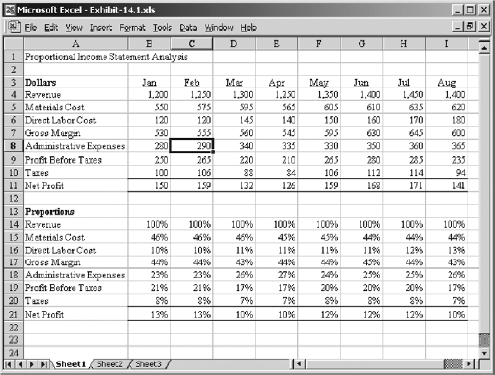For each line item in this top section, there is a formula entered in the replicated income statement at the bottom of the screen that divides each expense line item by the revenue figure, resulting in a percentage of sales for each item. For example: the materials cost proportion for the month of January is calculated with the following formula, which is entered in cell B15:

B5 / B\$4

Since the spreadsheet contains the income statement for multiple months, the resulting proportional analysis becomes very useful for finding any trends in the expenses being incurred over the course of the year.

The income statement proportional analysis used in the preceding example would be of great use to management in determining why its profits are not increasing along with its evident sales growth. In the example, sales increase from \$1,200 in January to \$1,400 in August, but profits drop by \$9. Why? By perusing the proportional analysis, it is an easy matter to see that the cost of materials has dropped as a percentage of sales, which may reflect excellent purchasing, design, or production work to lower these costs. For the answer to why profits have dropped, we must look lower in the spreadsheet. The direct labor cost as a proportion of sales has risen, so this is an obvious target area for further analysis. However, the overall gross margin percentage has only dropped by one percent over the time period being analyzed, so there must be more trouble further down in the income statement.

Sure enough, the administrative expenses line item reveals a three percent jump in costs. Accordingly, anyone using this analysis would conclude that the trouble has arisen in the direct labor and administrative areas, and that the materials expense requires no further analysis.

Though this type of analysis is an excellent way to hone in on key areas, it is rarely the final analysis conducted, since it does not reveal enough information. Also, it is not sufficient if there are many operating divisions rolled into the income statement. In these cases, it is best to create a number of separate spreadsheets, one for each division, and conduct the analysis on each one, thereby yielding a greater level of detail regarding problem areas.

The same proportional analysis can be applied to the balance sheet. In the next figure, one can manually enter a simplified version of the balance sheet at the top of the spreadsheet, which produces a set of percentages at the bottom. The asset percentages sum to the grand total of all assets, while the percentages for liabilities and equity sum to the total for those two categories.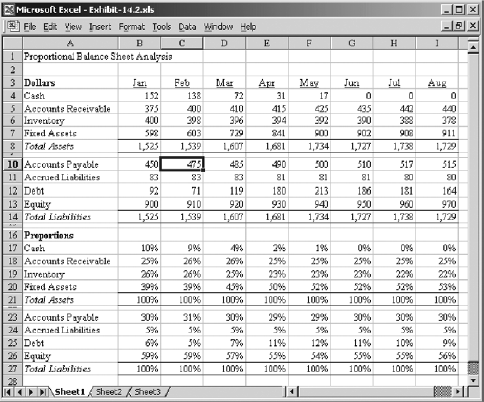As was the case for the proportional analysis of the income statement, the cell formula is extremely simple. In the above figure, the percentage for accounts payable in April is calculated by dividing the total accounts payable dollars, located in cell E10, by the total of all liabilities and equity for that month, which is located in cell E14.

What does the proportional analysis of the balance sheet tell us? To use the example, there is a clear increase in the fixed asset investment, which requires the use of all cash, as well as an increased debt load, which reaches its height in May, after which cash flow from operations is used to gradually draw down the level of debt. The only other trend of note is that inventory levels are declining, which indicates either excellent logistics practices or a decline in sales that no longer requires such a large supporting base of inventory. Consequently, a great deal can be discerned by reviewing a proportional balance sheet analysis.

Financial Statement Ratio Analysis

Perhaps the most common use of an electronic spreadsheet is to conduct a ratio analysis of the income statement and balance sheets. Typically, a summary form of the income statement and balance sheet are located at the top of the worksheet, with ratios located at the bottom that are derived from these two reports. By using this approach, one can quickly enter the summary-level financial information for the current reporting period and then see the related ratios appear at the bottom of the worksheet. In a few moments, he or she has access to a rough analysis of company operations. If there are entries for the financial results of previous months, then one can also see trend lines in ratio results that extend through to the current reporting period.

As an example of the types of ratio analysis one can use in a worksheet, we will use the “income statement and balance sheet” shown earlier, in the first and second screenshot above. A series of ratios are noted in below figure that are derived from those statements.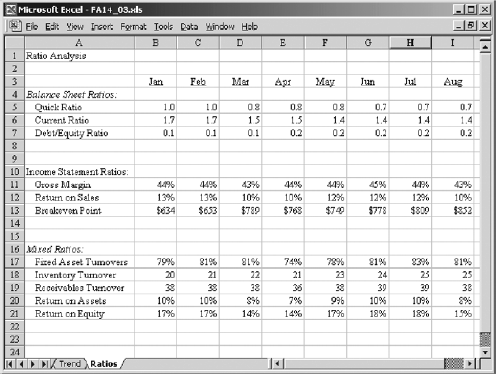In the above figure, there are several tabs itemized at the bottom of the worksheet. Each one represents another spreadsheet that is clustered into the same workbook. The first tab, entitled “IS“, contains a spreadsheet version of the income statement.

The second tab, entitled BS, contains a spreadsheet version of the balance sheet. The ratios shown in the Figure are compiled by referencing the cell locations in these two spreadsheets and listing the result on the current Ratios spreadsheet.

The formulas behind the ratios in the above figure are not shown, so the same spreadsheet is laid out differently in below figure to provide this information. In this example, we have eliminated the formulas for all but the month of January, and then listed each formula in full. For example, the first ratio is the Quick Ratio, which compares easily liquidated assets to current liabilities. To obtain this information, the cell entry goes to the “BS” spreadsheet and adds together cells B4 and B5, which contain the cash and accounts receivable figures for the month of January. The formula then divides the sum by the accounts payable and accrued liabilities amounts, which are located on the same spreadsheet in cells B10 and B11.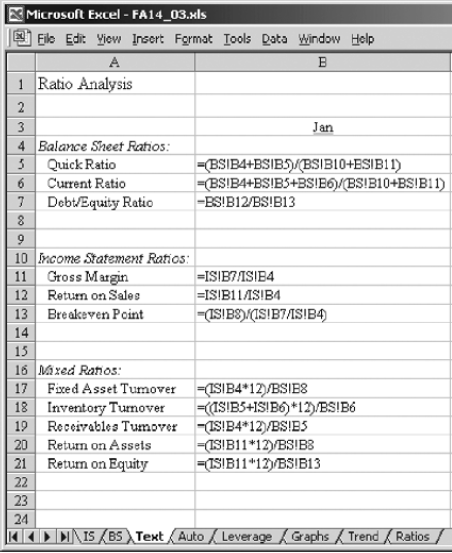Further down in the list of ratios are ones that are built upon the income statement. For example: the Return on Sales percentage is derived by referencing the profit figure for January, which is located in cell B11 in the IS spreadsheet and dividing by total sales, which is located in cell B4 in the same spreadsheet. Finally, we can mix references to both the IS and BS spreadsheets in the same ratio formula.

For example: mto arrive at the return on equity, the formula takes the profit for January, which is located in cell B11 in the IS spreadsheet, annualizes it by multiplying by 12, and divides it by the equity figure, which is located in cell B13 in the BS spreadsheet. Thus, we can mix cell references from a variety of spreadsheets in order to arrive at a centralized set of ratios that can be stored in a single spreadsheet location.

Automated Ratio Result Analysis

If there are a great many ratios linked to a set of financial statements, one may want to save time in reviewing them by having the spreadsheet issue a warning message for those ratios that fall outside a preset parameter. Another reason for using this approach is when a lending institution places constraints on a company by requiring minimum levels for certain ratios, such as a current ratio of at least 2:1, or a debt/equity ratio of no higher than 30%. In either case, a formula that presents a YES/NO or GOOD/BAD result can save some time.

A simple IF formula will create an automated ratio result. To continue with the example used previously in the above figure, we will add three rows to the analysis. Under the Balance Sheet Ratios section, add a row entitled “Meets Quick Ratio Covenant” This is a YES/NO determination based on the quick ratio being greater than 0.9, and will appear in row 8. The formula for the month of January will be:

IF(B5>.9,”Yes”,”No”)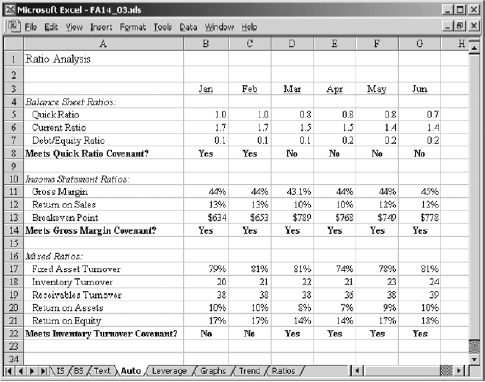Under the Income Statement Ratios section, add a row entitled Meets Gross Margin Covenant. This is a YES/NO determination based on the gross margin being greater than 43% and will appear in row 14. The formula for the month of January will be:

IF(B18>21,“Yes”,“No”)

All of these new formulations are shown in the ratios Figure on the next screenshot. In the Figure, one can quickly skim through the various months of results to determine the occasions when covenants have been violated. Setting up the IF statements that drive these automated ratio results are quite simple, and can help to some extent in the task of sorting through large quantities of ratios.

Are you looking for easy accounting tutorial? Established since 2007, Accounting-Financial-Tax.com hosts more than 1300 articles (still growing), and has helped millions accounting student, teacher, junior accountants and small business owners, worldwide.